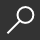## The Nature of Mathematical Modeling by Neil Gershenfeld

Overview -
This book first covers exact and approximate analytical techniques (ordinary differential and difference equations, partial differential equations, variational principles, stochastic processes); numerical methods (finite differences for ODE's and PDE's, finite elements, cellular automata); model inference based on observations (function fitting, data transforms, network architectures, search techniques, density estimation); as well as the special role of time in modeling (filtering and state estimation, hidden Markov processes, linear and nonlinear time series). Each of the topics in the book would be the worthy subject of a dedicated text, but only by presenting the material in this way is it possible to make so much material accessible to so many people. Each chapter presents a concise summary of the core results in an area, providing an orientation to what they can (and cannot) do, enough background to use them to solve typical problems, and pointers to access the literature for particular applications.

local_shippingFor DeliveryIn Stock.
This item is Non-Returnable.
FREE Shipping for Club Members helpNew & Used Marketplace 9 copies from \$50.72

## More About The Nature of Mathematical Modeling by Neil Gershenfeld

### Overview

This book first covers exact and approximate analytical techniques (ordinary differential and difference equations, partial differential equations, variational principles, stochastic processes); numerical methods (finite differences for ODE's and PDE's, finite elements, cellular automata); model inference based on observations (function fitting, data transforms, network architectures, search techniques, density estimation); as well as the special role of time in modeling (filtering and state estimation, hidden Markov processes, linear and nonlinear time series). Each of the topics in the book would be the worthy subject of a dedicated text, but only by presenting the material in this way is it possible to make so much material accessible to so many people. Each chapter presents a concise summary of the core results in an area, providing an orientation to what they can (and cannot) do, enough background to use them to solve typical problems, and pointers to access the literature for particular applications.

This item is Non-Returnable.

### Details

• ISBN-13: 9780521210508
• ISBN-10: 052121050X
• Publisher: Cambridge University Press
• Publish Date: June 2011
• Page Count: 358
• Dimensions: 9.61 x 6.69 x 0.74 inches
• Shipping Weight: 1.26 poundsRelated Categories